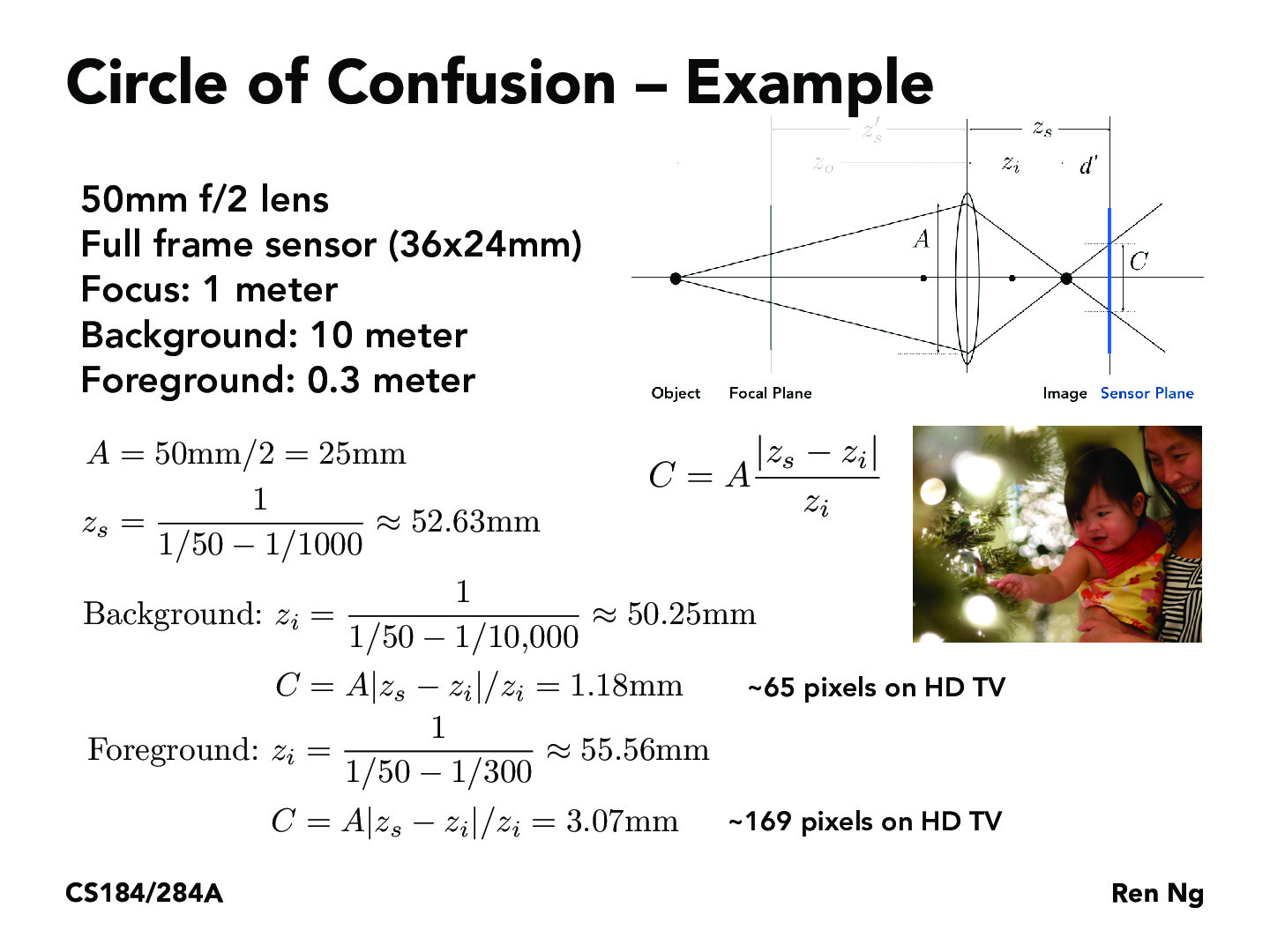You are viewing the course site for a past offering of this course. The current offering may be found here.Lecture 16: Cameras and Lenses (84)tsekylekobie

What's the difference between z_i and z_s? Looking at the diagram, I would have thought that z_i is the diagram focus because that's where the lines converge.jshergill426

Z_I is the distance where the Blur Circle of Diameter C is in the focused object plane, while Z_S is the distance where the same Blur Circle is an unfocused virtual image. https://en.wikipedia.org/wiki/Circle_of_confusionYanda-Li

I don't really understand the part of zs, where does that equation come from?

You must be enrolled in the course to comment